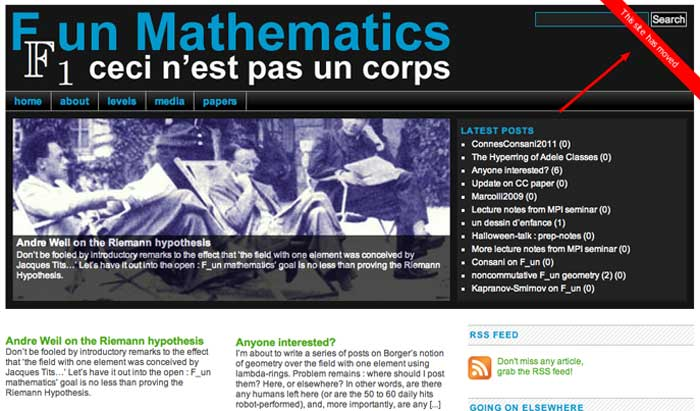For some time I knew it was in the making, now they are ready to launch it:

The $\mathbb{F}_1$ World Seminar, an online seminar dedicated to the “field with one element”, and its many connections to areas in mathematics such as arithmetic, geometry, representation theory and combinatorics. The organisers are Jaiung Jun, Oliver Lorscheid, Yuri Manin, Matt Szczesny, Koen Thas and Matt Young.

From the announcement:

“While the origins of the “$\mathbb{F}_1$-story” go back to attempts to transfer Weil’s proof of the Riemann Hypothesis from the function field case to that of number fields on one hand, and Tits’s Dream of realizing Weyl groups as the $\mathbb{F}_1$ points of algebraic groups on the other, the “$\mathbb{F}_1$” moniker has come to encompass a wide variety of phenomena and analogies spanning algebraic geometry, algebraic topology, arithmetic, combinatorics, representation theory, non-commutative geometry etc. It is therefore impossible to compile an exhaustive list of topics that might be discussed. The following is but a small sample of topics that may be covered:

Algebraic geometry in non-additive contexts – monoid schemes, lambda-schemes, blue schemes, semiring and hyperfield schemes, etc.
Arithmetic – connections with motives, non-archimedean and analytic geometry
Tropical geometry and geometric matroid theory
Algebraic topology – K-theory of monoid and other “non-additive” schemes/categories, higher Segal spaces
Representation theory – Hall algebras, degenerations of quantum groups, quivers
Combinatorics – finite field and incidence geometry, and various generalizations”

The seminar takes place on alternating Wednesdays from 15:00 PM – 16:00 PM European Standard Time (=GMT+1). There will be room for mathematical discussion after each lecture.

The first meeting takes place Wednesday, January 19th 2022. If you want to receive abstracts of the talks and their Zoom-links, you should sign up for the mailing list.

Perhaps I’ll start posting about $\mathbb{F}_1$ again, either here, or on the dormant $\mathbb{F}_1$ mathematics blog. (see this post for its history).